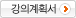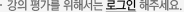## 주메뉴

### Educational Evaluation Theory (Item Response Theory)

• 부산대학교
• 김석우
• 주제분류
교육학 >교육일반 >교과과정
• 강의학기
2018년2학기
• 조회수
829
•
강의계획서This course aims to help the educaion majors understand the basic concepts of test and measurement and to introduce the Classical Test Theory and the new theories relating to the Item Response Theory.

#### 차시별 강의1Test Theory 1. Know the concept of Classical Test Theory. 2. Know the concept of Item Response Theory. 3. Differences between classical test theory and item response theory.2Concepts, Models, and Features 1. Understand the assumptions of IRT.                               2. Know one-, two-, and three-parameter logistic models.3. Know the Property of Invariance.3ltem Parameter and Ability Estimation 1. Know how to estimate the ability and item parameters.2. Understand the standard errors of item parameter estimates.4Assessment of Model-Data Fit 1. Know the limitation of goodness-of-fit studies.2. Understand the judgment about the fit based on 3 types of evidence5The Ability Scale and True Score 1. Understand the nature of the ability scale. 2. Know how to transform the theta scale.6Item and Test Information and Efficiency Funcitons 1. Understand the basic concepts of item information functions. 2. Understand the basic concepts of test information functions.7Test Construction 1. Know the test construction approach in CTT. 2. Know the test construction approach in IRT.8Identification of Potentially Biased Test Items 1. Understand the concept of differential item functioning. 2. Know the IRT methods for detecting differential item functioning.9Test Score Equating 1. Know the concept of test score equating.2. Understand the classical methods of equating.3. Understand the IRT methods of equating.10Specifying the Characteristics of a test 1. Know the concept of screening tests.2. Know the concept of broad-ranged tests and peaked tests.3. Know the concept of computer Adaptive Test#### 연관 자료#### 사용자 의견#### 이용방법

• 문서 자료 이용시 필요한 프로그램 [바로가기]

※ 강의별로 교수님의 사정에 따라 전체 차시 중 일부 차시만 공개되는 경우가 있으니 양해 부탁드립니다.